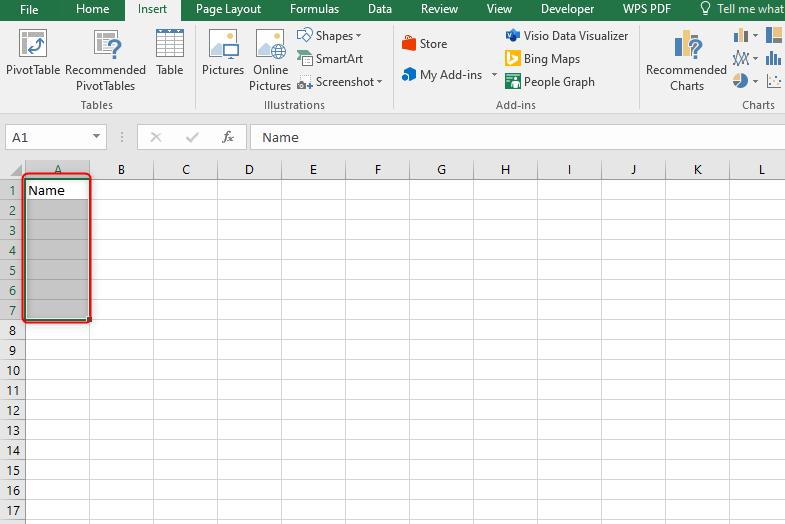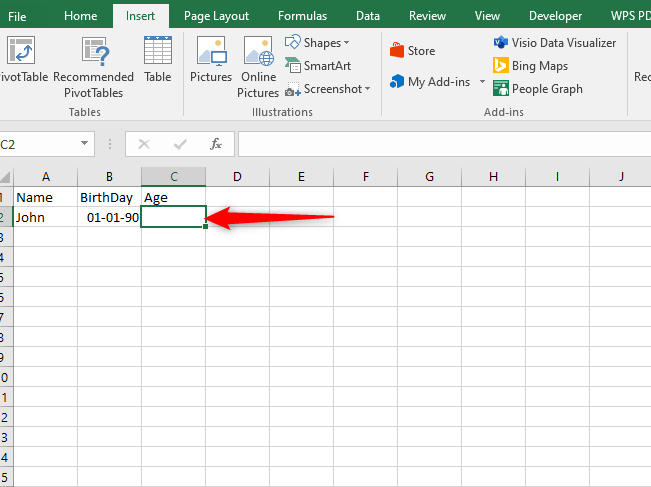# How to Calculate Age Using Excel

Have you ever wondered how you could calculate the age of a number of people in a short period of time? Well, excel simplifies this task for you. Since calculating age goes hand in hand with dates and numbers in excel, it is easier to calculate age when you follow a few steps. It is important to know the date of birth of the person when calculating one's age. The following steps  guide in calculating age in excel:

1. Create a Name column

It isn't compulsory to have the title 'Name' but bear in mind that this column will help identify each person you are calculating the birthday for2. Create a Birthday column.

This column can contain every birthday as a separate line. It isn't a must you use this for birthdays only you can choose any starting point such we purchased date3. Enter the birthdays using a common format.

The format could be either MM/DD/YYYY normally for US citizens or DD/MM/YYYY for non-US residents.

• If the data is being auto-updated as something else, highlight the cells and click a drop-down menu in the Numbers section of the Home tab then select a short date from the list of options4. Create an age column

This will display the age for each entry after you enter the formula5. Select the primary empty cell in the Age column. This is where you will be entering the formula to calculate birthdays6. Enter the formula for finding the age in years

=DATED IF (B2,TODAY ( ), "Y" )Whereby:

=DATED IF ( ) is a function that calculates the difference between the two dates

( B2,TODAY ( ), "Y" ) tells DATED IF to calculate the distinction between the date in cell B2 (the initial birthday listed ) and the current date ( nowadays( ) )

To see age in days or months use "D" or "M" instead.

7. Click and drag the square within the bottom right corner of the cell down. This may apply an equivalent formula to every line, adjusting it consequently so that the proper birthday is calculated.8. Modify the formula to calculate exact age in years , months and days .The advanced formula is

=DATED IF ( B2, TODAY ( ), "Y" )&years, "&DATED IF (B2,TODAY ( ), "Y") & "months, "& DATED IF (B2,TODAY ( ), "MD" ) & "Days"

Note that the DATED IF formula does not work for dates before 01/01/1900

Alternatively, EDUCBA.com gives a simplified formula for calculating age.

(B1-B2)/365.25

Whereby:

B1= current date

B2= date of birth

365.25= number of days in a yearTo round off the decimal place in 365.25 use the ROUND DOWN function formula

=ROUNDDOWN ( Number, num-digit)

The number is used to round down the number and the num-digit is used to round down the number to many decimal places. Then apply the formula

=ROUNDDOWN ((B1-B2)/365.25,0)## Applying The Basic TODAY Function

The simplest way to determine someone's age is by subtracting the birthday date from the current date. That means you can also apply this basic formula in Excel following these steps:

1. Select the cell next to the birthday and write the formula below. For example, you can select cell D5 when cell C5 is the birthday column.

=(TODAY()-C5)/365.252. Click the Enter button. Here, the TODAY function returns today’s date. We also divide the differences with 365.25 because one year consists of 365 days but a leap year comes after four years.

3. Use the Fill Handle to drag the formula down column D. You will get the age for each birthday displayed in column D.4. If you want to see the age in complete years, you can apply the INT function. In this case, write the formula in cell E5. However, ensure cell range or column D5 is formatted in Number or General.

=INT(D5)## Combining IF, YEAR, MONTH, and NOW Functions

Each function in the combination has its role in ensuring you get the age from someone’s birthday. Here, you can follow these steps:

1. Write the formula below in the cell next to the birthday column. For example, you can select cell D5 if the birthdays are recorded in column C.

=IF(MONTH(NOW())>MONTH(C5),YEAR(NOW())-YEAR(C5)&"yr "&MONTH(NOW())-MONTH(C5)&"mo",YEAR(NOW())-(YEAR(C5)+1)&"yr "&(MONTH(NOW())+12)-MONTH(C5)&"mo")The MONTH and YEAR functions will return the month and year of the current date as a number. On the other hand, the IF function gives a logical test that, if the month of the current date is greater than the month of the birthdate, then the formula will be:

=YEAR(NOW())-YEAR(C5)&"yr "&MONTH(NOW())-MONTH(C5)&"mo"

Therefore, the general formula will be;

=YEAR(NOW())-(YEAR(C5)+1)&"yr "&(MONTH(NOW())+12)-MONTH(C5)&"mo"## Applying the YEARFRAC Function

The YEARFRAC function returns a fraction of the year, though it is also a reliable method to use when calculating age from birthdays. It calculates the difference between the birthdate and the present date.

1. Select the cell where you want to display the result. You can select cell D5 if the birthdays are recorded in column C.

2. Write the following formula in the selected cell and click the Enter button:

=YEARFRAC(C5,TODAY(),1)

where:

1 is counted as the basis or actual.3. If you want to get the age in full years, you can apply the INT function in cell E5 as follows;

=INT(D5)## Combining IF And DATE IF Functions to Get Only Non-Zero Values

When using the DATE IF Function, you are likely to get zero months and zero days in some cells. Therefore, to avoid displaying zero values, you can introduce the IF function to get an updated formula. Here, you can use these steps:

1. Select the cell next to the birthday column, say cell D5, and write the following formula:

=IF(DATEDIF(C5, TODAY(),"y")=0,"",DATEDIF(C5, TODAY(),"y")&" years, ")& IF(DATEDIF(C5, TODAY(),"ym")=0,"",DATEDIF(C5, TODAY(),"ym")&" months, ")& IF(DATEDIF(C5, TODAY(),"md")=0,"",DATEDIF(C5, TODAY(),"md")&" days")

The formula works similarly to the DATE IF function, though it omits any zero value found in years, months, and days.2. Use the Fill Handle to drag the formula down the column.## Using VBA Code

You can also use the VBA code to calculate age from birthday in Excel as follows:

1. Right-click on the Sheet Name and click on View Code.2. When the Microsoft Visual Basic for Applications window opens, right-click on Sheet9 (VBA) and select Insert. Finish by clicking on Module.3. A code module will open. Copy and paste the code below and click on the Run button. You can also press the F5 to run the code.

Sub Age()

Dim LastRow As Long

With ThisWorkbook.Worksheets("VBA")

LastRow = .Cells(Rows.Count, "C").End(xlUp).Row

.Range("E5:E" & LastRow) = "=DATEDIF(C5,Now(),""y"")"

End With

End Sub4. Close the code module and go back to the worksheet. You will see the cell range, say D5:D10 filled with the age in years.